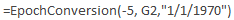I ran into an interesting problem earlier today. I was using a CDR dump from our Cisco system. However, all of the datetime fields were represented as seconds. I knew right away that it used an epoch value. What I didn’t know is what the originating date was. Apparently, I was correct at my first guess. I guessed that it was using the standard Unix epoch value of 1/1/1970. Many applications, such as Microsoft Excel, use 1/1/1900.

Since I wanted to represent the correct date and time in Excel, I began looking around for a formula. To my surprise, nothing exists out of the box (unless my eyes are failing me). So, I had two options. The first option I headed down had me save my spreadsheet as a macro-enabled workbook. From there, I added a formula to an empty module.

``````1
2
3
Public Function EpochConversion(timeZoneOffset As Long, myNumber As Long, myDate As Date) As Date
EpochConversion = DateAdd("s", myNumber + (timeZoneOffset * 3600), myDate)
End Function
``````

The function accepts 3 parameters: timeZoneOffset which is the offset from GMT (for example, New York City is in the Eastern Time Zone which is –5), the number of seconds, and the base date (in the event I need to use 1/1/1900 instead). So, an example use would look like this:That works, but it requires that my spreadsheet is a macro-enabled workbook. I can accomplish something similar by using the following formula. This is my second option for handling the conversion.

``````1
=(((G2-(5*3600))/86400)+25569)
``````

In this formula, the following values are used:

 G2 The cell containing the number of seconds from epoch. - If you are in the western hemisphere, this would be negative. Otherwise, change this to a plus sign. This handles the offset from GMT. 5 The is the value of the timezone from GMT. It's combined with the minus sign above. 3600 The number of seconds in an hour used to assist with the timezone offset. 86400 The number of seconds in a day. 25569 The number of days since 1/1/1900 to assist Excel in the proper epoch conversion of 1/1/1970.

In either case, I’ll obtain the same result.

One thing is for sure, I’m hoping that I’m retired by January 19th, 2038 so that we don’t have another Y2K crisis when the 32-bit versions of epoch have overflows: http://2038bug.com/.